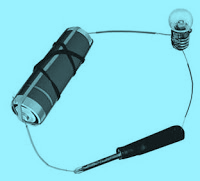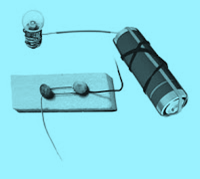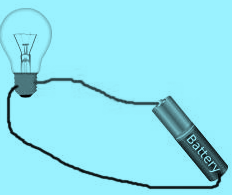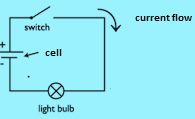# NCERT Solutions for Class 6 Science Chapter 12 Electricity and Circuits

In this page we have NCERT Solutions for Class 6 Science Chapter 12 Electricity and Circuits . Hope you like them and do not forget to like , social share and comment at the end of the page.
Question 1
Fill in the blanks :
(a) A device that is used to break an electric circuit is called ____________ .
(b) An electric cell has __________ terminals.
(c) Electric cell is a device which converts energy of chemicals into ______energy.
(d) The electric energy which is supplied in our home comes from electric__________houses.
(e) An electric _______ is a continuous path along which the current flows.
(f) If the filament of a bulb breaks, it is said to be ______.
(g) Rubber is a good example of electric ___.
(a) Switch
(b) 2- terminals
(c) Electrical
(d) poles nearby
(e) circuit
(f) fused
(g) Insulator

Question 2
Mark True or False for following statements:
(a) Electric current can flow through metals.
(b) Instead of metal wires, a jute string can be used to make a circuit.
(c) Electric current can pass through a sheet of thermocol.
(d) When current flows through a circuit, the circuit is called open circuit.
(e) An electric bulb has one terminal.
(a) True
(b) False
(c) false
(d) false
(e) false
Question 3
What is an electric cell?
(a) An electric cell is a device which converts chemical energy into electric energy.
(b) It has two different metal plates:
• one is the positive terminal
• the other is the negative terminal
(c) These plates are kept inside a chemical called electrolyte solution, which stores the chemical energy.

Question 4
Explain why the bulb would not glow in the arrangement shown in Figure below:The answer is No as the handle of the screw driver acts as an insulator. It does not allow current to flow and circuit is still open. So the bulb does not glow in the arrangement.

Question 5
Complete the drawing shown in Figure below to indicate where the free ends of the two wires should be joined to make the bulb glow.a. The bulb will glow when circuit is complete.
b. Now to complete the circuit, we need to connect one end of the wire from clip to positive terminal.
c. Second end of the wire from the clip need to be connected to the bulb.
d. Once all these connections are completed, The bulb will glow.
Question 6
Would the bulb glow after completing the circuit shown in Fig. above (Question 5) if instead of safety pin we use an eraser?

The answer would be No as eraser is made of rubber which is a poor conductor of electricity i.e. it is insulator. Therefore, bulb would not glow.
Question 7
What is the purpose of using an electric switch? Name some electrical gadgets that have switches built into them.
What is an electric switch?
An electric switch is a simple device which is used to break or complete an electric circuit.
Switches are widely used in different electrical or electronic gadgets. A few are:
a. Toggle switches used in houses.
b. Push button switches used in microwaves, water pump, mixer etc.
Question 8
Do all materials allow electricity to pass through them?
No. We have lot of material which does not allow electricity

Question 9
Would the bulb glow in the circuit shown in Figure below?The electric bulb has two terminals both the wires are connected to the one terminal of the bulb. Current will not flow from the bulb and it will not glow. To make glow, we have to connect two wires to each terminal of the bulb
Question 10
What are the components of an electric circuit?
In general, a circuit has the following components:
1. A cell or battery which is the source of electricity. We could have one cell or more cell
2. Connecting wires by which you will connect the electric cell to the electric device
3. Bulb or electric device l
4. Electric switch.
Example electric circuit### Practice Question

Question 1 What is $\frac {1}{2} + \frac {3}{4}$ ?
A)$\frac {5}{4}$
B)$\frac {1}{4}$
C)$1$
D)$\frac {4}{5}$
Question 2 Pinhole camera produces an ?
A)An erect and small image
B)an Inverted and small image
C)An inverted and enlarged image
D)None of the above